• Communicate the findings to people.
• Using python for best practice to visualize data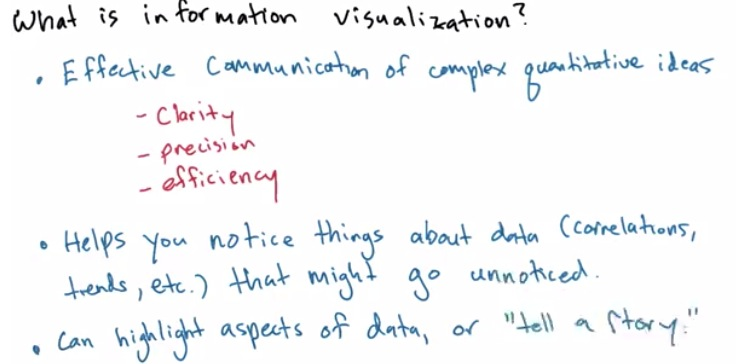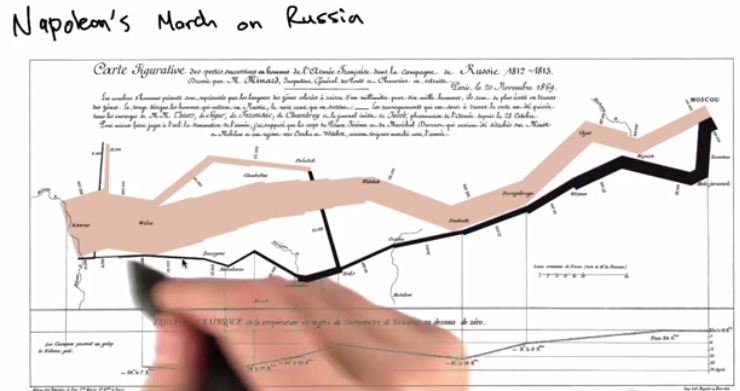• This is the graph represent the title
• Brown is the color which invades to rusia, and black is the color which return home from rusia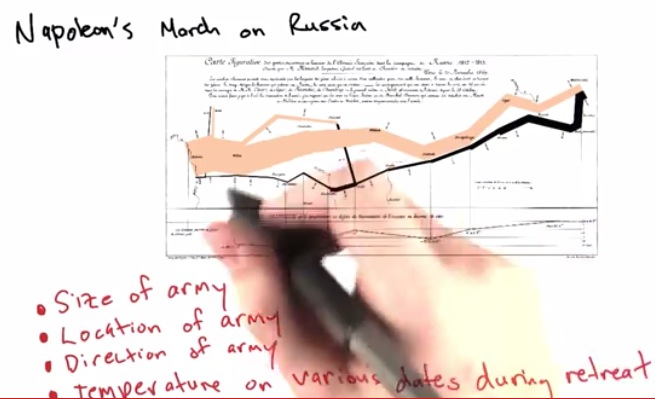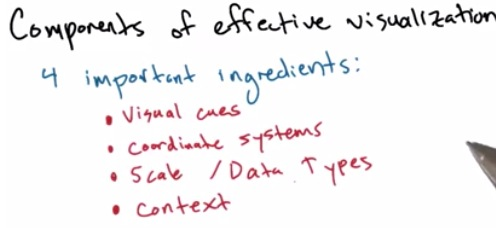• These are the factors to create effective visualization
• Now, take baseball charts for example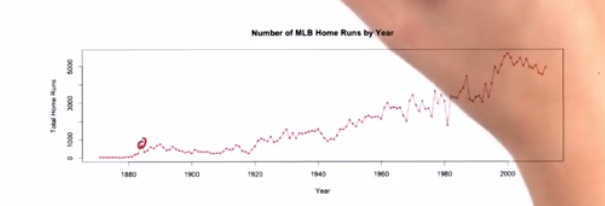• Visual Cues/Visual Encoding: Endoding with color/type/size. By connecting the dot. We can see the increase/decrease of the data
• Coordinate Systems: Here we implement y-axis to represent years, and x-axis represent how much the home run. So from the table here, we can say that how many homerun hit over the years
• Scale/Data Types: Represent how we scale/type our data. Divided by three parts, numeric, category, time. x-axis represent numeric based, while the years represent time-based. Also the scale is linear type, with increasing. other scale discussed later
• Context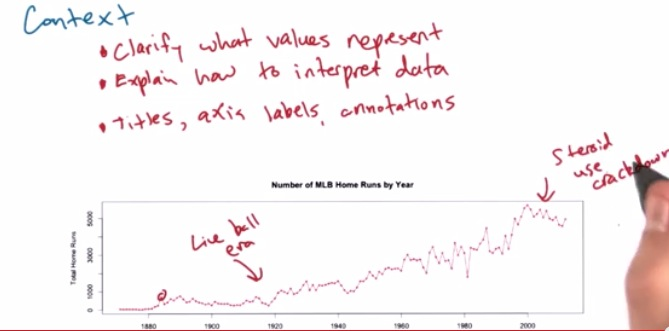• Based on visualization, it's our job to represent how people read our data.
• How we explain the value in the table.
• In doing so, we can make all the labels(title,axis etc). Also annotate what can be pointed out from the graph(e.g. live bell era in years 1920, streoid use around 2007)